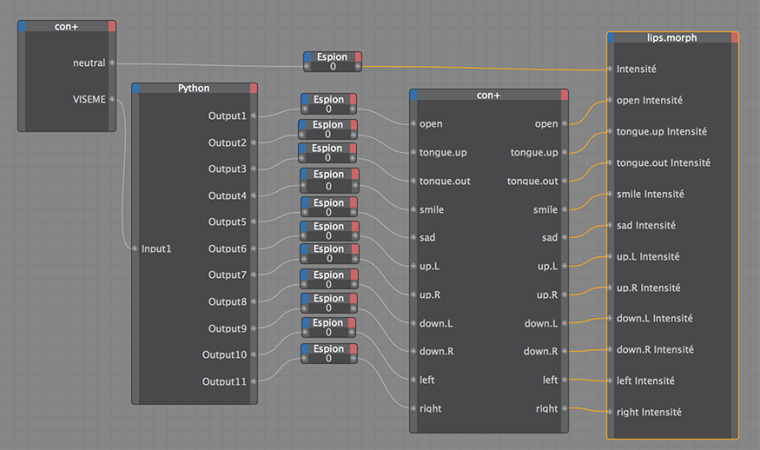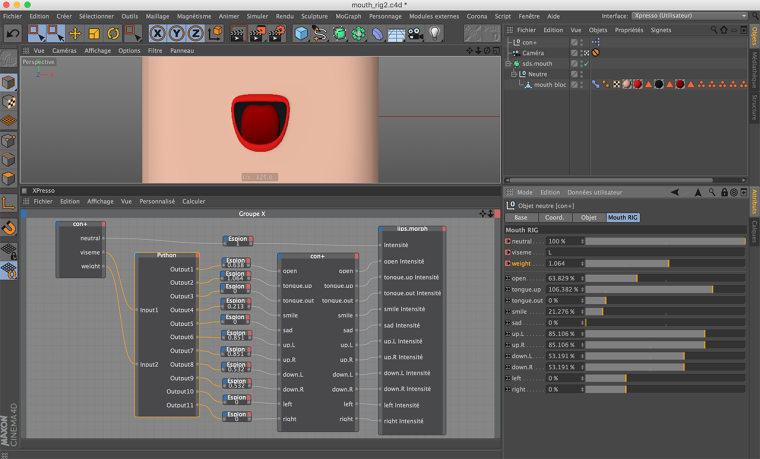# Viseme RIG test

• Hello,

in order to faster the mouth animation process of a character,
i try to create a viseme rig.

I have put on an object a pose morph tag with 6 sliders for 6 visemes.
I created a dynamic text in user data, to edit viseme orders .
I created an Xpresso tag, with python node, to control visemes sliders.
It works, but in a boolean way !

I want to improve this Xpresso and python Rig to control sliders
in a linear way (from 0% to 100%, or 100% to 0%) !
With duration for the moving sliders between viseme orders
With conditions for the position of the sliders (e.g.: if slider 1 at position 0, go to 100, if slider 2 at position 100, go to 0 ....).

How can i do it ?
I'm a python rookie !

Thank's

• Hi Gal, thanks for writing us.

With regard to the issue reported, it looks to me like some sorting of unwanted data-clamping effect due to type casting. To be more helpful please provide your code snippet ( or a complete test asset) in order to dig further.

Best, Riccardo

• @r_gigante said in Viseme RIG test:

With regard to the issue reported, it looks to me like some sorting of unwanted data-clamping effect due to type casting. To be more helpful please provide your code snippet ( or a complete test asset) in order to dig further.

Hi Ricardo,

I've a little bit improved my xpresso nodes, simple but efficient !The viseme are controlled in a boolean way = step key frame.
With controlling the global intensity of the pose morph tag,
i can have more interpolation of the lips movement between the viseme step keys frames.
Mouth rig test_animatic

How to improved python code in order to have viseme linear key frames ?

Thanks
Best regards
Gal

This is my simple python code :

``````import c4d
#Welcome to the world of Python

def main():
global Output1, Output2, Output3, Output4,  Output5,  Output6,  Output7,  Output8,  Output9,  Output10,  Output11

if Input1 == "":
Output1 = 0
Output2 = 0
Output3 = 0
Output4 = 0
Output5 = 0
Output6 = 0
Output7 = 0
Output8 = 0
Output9 = 0
Output10 = 0
Output11 = 0

if Input1 == "E":
Output1 = 1
Output2 = 0
Output3 = 0
Output4 = 0
Output5 = 0
Output6 = 0
Output7 = 0
Output8 = 0
Output9 = 0
Output10 = 0
Output11 = 0

if Input1 == "A":
Output1 = 0.8
Output2 = 0
Output3 = 0
Output4 = 0.8
Output5 = 0
Output6 = 0
Output7 = 0
Output8 = 0
Output9 = 0
Output10 = 0.5
Output11 = 0.5

if Input1 == "I":
Output1 = 0
Output2 = 0
Output3 = 0
Output4 = 1
Output5 = 0
Output6 = 1
Output7 = 1
Output8 = 0
Output9 = 0
Output10 = 0.5
Output11 = 0.5

if Input1 == "U":
Output1 = 0.8
Output2 = 0
Output3 = 0
Output4 = 0
Output5 = 0
Output6 = 0
Output7 = 0
Output8 = 1
Output9 = 1
Output10 = 0
Output11 = 0

if Input1 == "R":
Output1 = 0.5
Output2 = 0
Output3 = 0
Output4 = 1
Output5 = 0
Output6 = -0.5
Output7 = -0.5
Output8 = 1
Output9 = 1
Output10 = 0.5
Output11 = 0.5

if Input1 == "O":
Output1 = 0.9
Output2 = 0
Output3 = 0
Output4 = 0
Output5 = 0
Output6 = 0
Output7 = 0
Output8 = 0.4
Output9 = 0.4
Output10 = -0.3
Output11 = -0.3

if Input1 == "CH":
Output1 = 0.2
Output2 = 0
Output3 = 0
Output4 = 0.35
Output5 = 0
Output6 = 0.5
Output7 = 0.5
Output8 = 0
Output9 = 0
Output10 = 0.6
Output11 = 0.6

if Input1 == "S":
Output1 = 0.1
Output2 = 0
Output3 = 0
Output4 = 0.41
Output5 = 0.2
Output6 = 1
Output7 = 1
Output8 = 1
Output9 = 1
Output10 = 1
Output11 = 1

if Input1 == "M":
Output1 = 0
Output2 = 0
Output3 = 0
Output4 = 0.1
Output5 = 0.3
Output6 = 0.6
Output7 = 0.6
Output8 = 0.85
Output9 = 0.85
Output10 = 0.4
Output11 = 0.4

if Input1 == "D":
Output1 = 0.05
Output2 = 0
Output3 = 0
Output4 = 0.3
Output5 = 0.2
Output6 = 0.8
Output7 = 0.8
Output8 = 0.85
Output9 = 0.85
Output10 = 0.2
Output11 = 0.2

if Input1 == "F":
Output1 = 0.05
Output2 = 0
Output3 = 0
Output4 = 0
Output5 = 0.2
Output6 = 0.8
Output7 = 0.8
Output8 = 0.5
Output9 = 0.5
Output10 = 0
Output11 = 0

if Input1 == "L":
Output1 = 0.6
Output2 = 1
Output3 = 0
Output4 = 0.2
Output5 = 0
Output6 = 0.8
Output7 = 0.8
Output8 = 0.5
Output9 = 0.5
Output10 = 0
Output11 = 0``````

• Hi Gal, thanks for following-up.

With regard to your setup, without changing too much things, you could simply consider to add an additional float parameter to your VISEME data - in order to have three input params "neutral", "VISEME" and a "weight" - and use its value defined by a curve in your python script (below to keep your naming scheme is called Input2) in order to change the weight of your VISEME rig.

``````import c4d

def main():

global Output1, Output2, Output3, Output4,  Output5,  Output6,  Output7,  Output8,  Output9,  Output10,  Output11

# Input2 is the float parameter defined to weight the viseme
Output1 = 0
Output2 = 0
Output3 = 0
Output4 = 0
Output5 = 0
Output6 = 0
Output7 = 0
Output8 = 0
Output9 = 0
Output10 = 0
Output11 = 0

if Input1 == "E":
Output1 = 1.0 * Input2
elif Input1 == "A":
Output1 = 0.8 * Input2
Output4 = 0.8 * Input2
Output10 = 0.5 * Input2
Output11 = 0.5 * Input2
elif Input1 == "I":
Output4 = 1.0 * Input2
Output6 = 1.0 * Input2
Output7 = 1.0 * Input2
Output10 = 0.5 * Input2
Output11 = 0.5 * Input2
elif Input1 == "U":
Output1 = 0.8 * Input2
Output8 = 1.0 * Input2
Output9 = 1.0 * Input2
elif Input1 == "R":
Output1 = 0.5 * Input2
Output4 = 1.0 * Input2
Output6 = -0.5 * Input2
Output7 = -0.5 * Input2
Output8 = 1.0 * Input2
Output9 = 1.0 * Input2
Output10 = 0.5 * Input2
Output11 = 0.5 * Input2
elif Input1 == "O":
Output1 = 0.9 * Input2
Output8 = 0.4 * Input2
Output9 = 0.4 * Input2
Output10 = -0.3 * Input2
Output11 = -0.3 * Input2
elif Input1 == "CH":
Output1 = 0.2 * Input2
Output4 = 0.35 * Input2
Output6 = 0.5 * Input2
Output7 = 0.5 * Input2
Output10 = 0.6 * Input2
Output11 = 0.6 * Input2
elif Input1 == "S":
Output1 = 0.1 * Input2
Output4 = 0.41 * Input2
Output5 = 0.2 * Input2
Output6 = 1.0 * Input2
Output7 = 1.0 * Input2
Output8 = 1.0 * Input2
Output9 = 1.0 * Input2
Output10 = 1.0 * Input2
Output11 = 1.0 * Input2
elif Input1 == "M":
Output4 = 0.1 * Input2
Output5 = 0.3 * Input2
Output6 = 0.6 * Input2
Output7 = 0.6 * Input2
Output8 = 0.85 * Input2
Output9 = 0.85 * Input2
Output10 = 0.4 * Input2
Output11 = 0.4 * Input2
elif Input1 == "D":
Output1 = 0.05 * Input2
Output4 = 0.3 * Input2
Output5 = 0.2 * Input2
Output6 = 0.8 * Input2
Output7 = 0.8 * Input2
Output8 = 0.85 * Input2
Output9 = 0.85 * Input2
Output10 = 0.2 * Input2
Output11 = 0.2 * Input2
elif Input1 == "F":
Output1 = 0.05 * Input2
Output5 = 0.2 * Input2
Output6 = 0.8 * Input2
Output7 = 0.8 * Input2
Output8 = 0.5 * Input2
Output9 = 0.5 * Input2
elif Input1 == "L":
Output1 = 0.6 * Input2
Output2 = 1.0 * Input2
Output4 = 0.2 * Input2
Output6 = 0.8 * Input2
Output7 = 0.8 * Input2
Output8 = 0.5 * Input2
Output9 = 0.5 * Input2
``````

Hoping it make sense, feel free to get back for any further help.

Cheer, Riccardo

• Hello

Thank you Ricardo for the code and this option.
It works for me !Is there a way to control an interpolation of data (e.g. 0 to 100) on a duration (e.g. 10 frames) with python, with only one order keyframe ?

If you have an advice or a weblink to better learn python for C4D, i'll take it !

Thanks

Gal

• Hi! You could go for CTracks via Python. You'd have to get the track for a position parameter, for example, or create one, then get curve, then add keys. Later, you could simply range map it back to your Xpresso rig. it It's definitely doable!